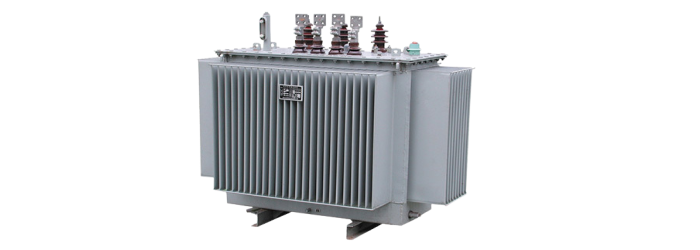# Why Are Transformers Rated in kVA, and not in kW?

Transformers are always rated in kVA instead of kW. Let's see why it is so.## Reason 1

The first reason is the power loss in the transformer. As, the copper loss or I2R loss depends on the current and the iron or core loss depends upon the voltage of the transformer. Thus, the total losses in a transformer depend upon volt-ampere (VA) only and not on the power factor of the load. That is why the transformer rating is given in kVA and not in kW.

## Reason 2

At the time of designing a transformer, the manufacturer does not know which kind of load (inductive or capacitive or resistive) will be connected in future. Also, the power factor depends upon the load. In actual practice, the pure inductive (lagging) and pure capacitive (leading) loads do not exist, i.e., every load has some resistance even it is inductive or capacitive. Hence, the practical loads draw both active and reactive powers from the supply.

For example, an induction motor has windings which are inductive and also possess some resistance. Thus, it draws both active power (kW) and reactive power (kVAR) so that the total power drawn by the motor is the phasor sum of kW and kVAR, which is equal to kVA. Also, the current drawn by the load depends upon the power factor of it, which is not determinant to the manufacturer at the time of designing. Due to this reason a transformer is always rated in kVA so that it can be used for any kind load (lagging or leading).

## Reason 3

The temperature rise of a transformer is directly proportional to the apparent power (kVA) which flows through it. This is also a principle factor behind that the rating of the transformer is given in kVA and not in kW.

Important – In actual practice, those electrical devices which act as the source (such as alternator, transformer) always rated in kVA to make their operation independent of the load power factor while the electrical devices which act as the load (e.g., motors) always rated in kW and draw the current from the source according to their power factor.